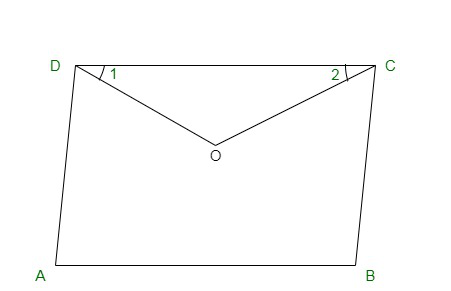Related Articles

# Class 9 RD Sharma Solutions – Chapter 14 Quadrilaterals- Exercise 14.1

• Last Updated : 08 Dec, 2020

### Question 1. Three angles of a quadrilateral are respectively equal to 110°, 50° and 40°. Find its fourth angle.

Solution:

Given,
The three angles are 110°, 50° and 40°
Let the fourth angle be ‘x’
Sum of all angles of a quadrilateral = 360°
110° + 50° + 40° + x = 360°
200° + x = 360°
x = 360° − 200°
x = 160°
Hence, the required fourth angle is 160°.

### Question 2. In a quadrilateral ABCD, the angles A, B, C and D are in the ratio of 1:2:4:5. Find the measure of each angles of the quadrilateral.

Solution:

Let the angles of quadrilateral be
A = x, B = 2x, C = 4x, D = 5x
Then,
A + B + C + D = 360° {Sum of interior angle of quadrilateral 360°}
x + 2x + 4x + 5x = 360°
12x = 360°
x = 360°
12
x = 30°
Therefore, substituting value of x,
A = x = 30°
B = 2x = 2 × 30° = 60°
C = 4x = 4 × 30° = 120°
D = 5x = 5 × 30° = 150°

### Question 3. In a quadrilateral ABCD, CO and Do are the bisectors of ∠C and ∠D respectively. Prove that ∠COD = 1/2 (∠A and ∠B)

Solution:In ΔDOC,
∠CDO + ∠COD + ∠DCO = 1800 [Angle sum property of a triangle]
or
1 ∠CDA + ∠COD +  1 ∠DCB = 1800
2                               2
∠COD = 1800 –  1 (∠CDA + ∠DCB) —->(equation 1)
2
Also
We know, sum of all angles of a quadrilateral = 360°
∠CDA + ∠DCB = 3600 – (∠DAB + ∠CBA) —->(equation 2)
Substituting equation 1 and equation 2
∠COD = 1800 –  1 {3600 – (∠DAB + ∠CBA)}
2
We can also write,
∠DAB = ∠A and ∠CBA = ∠B
∠COD = 1800 − 1800 + 1/2(∠A + ∠B))
∠COD = 1/2(∠A + ∠B)
Hence, Proved.

### Question 4. The angles of a quadrilateral are in the ratio 3:5:9:13. Find all the angles of the quadrilateral.

Solution:

Let the angles of quadrilateral be
A = 3x, B = 5x, C = 9x, D = 13x
Then,
A + B + C + D = 360° {{Sum of interior angle of quadrilateral 360°}
3x + 5x + 9x + 13x = 360°
30x = 360°
x =  360°
30
x = 12°
Therefore, substituting value of x,
A = 3x = 3×12 = 36°
B = 5x = 5×12° = 60°
C = 9x = 9×12° = 108°
D = 13x = 13×12° = 156°

Attention reader! Don’t stop learning now. Participate in the Scholorship Test for First-Step-to-DSA Course for Class 9 to 12 students.

My Personal Notes arrow_drop_up